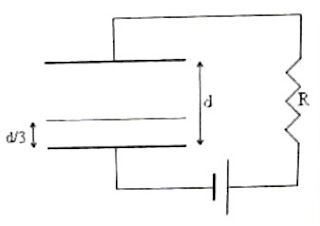## Wednesday, June 11, 2008

### IIT-JEE 2008 Multiple Choice Questions on Electrostatics

The following two questions from Electrostatics were included under Straight Objective Type (Multiple choice, single answer type) in the IIT-JEE 2008 question paper:

(1) Consider a system of three charges q/3, q/3 and –2q/3 placed at points A, B and C respectively as shown in the figure. Take O to be the centre of the circle of radius R and angle CAB = 60º(a) The electric field at point O is q/8πε0R2

(b) The potential energy of the system is zero

(c) The magnitude of the force between the charges at C and B is q2/54πε0R2

(d) The potential at point O is q/12πε0R

The electric field at O is due to the negative charge at C only since the equal positive charges situated at A and B will produce equal and opposite fields at O (and they will mutually cancel). The field at O is therefore negative and the option (a) is obviously wrong. Option (b) also is obviously wrong.

The magnitude of the force (F) between the charges at C and B is given by

F = (1/4πε0) [(2q/3)(q/3)/(2Rsin60º)2].

Thus F = q2/54πε0R2 as given in option (c).(2) A parallel plate capacitor with plates of unit area and separation d is filled with a liquid of dielectric constant K = 2. The level of liquid is d/3 initially. Suppose the liquid level decreases at a constant speed V. The time constant as a function of time t is

(a) 6ε0R/(5d + 3Vt)

(b) (15d + 9Vt) ε0R/(2d2 – 3dVt – 9V2t2)

(c) 6ε0R/(5d 3Vt)

(d) (15d – 9Vt) ε0R/(2d2 + 3dVt – 9V2t2)

When a dielectric slab of thickness t1 and dielectric constant K is introduced in between the plates of a parallel plate air capacitor of plate area A and plate separation d, the effective capacitance changes from ε0A/d to ε0A/[dt1 + (t1/K)]

The effective capacitance C of a parallel plate capacitor with parallel dielectric slabs of thickness t1, t2, t3 etc. of dielectric constants K1, K2, K3 etc. respectively is given by the series combined value of capacitors with these dielectrics. Therefore,

1/C = 1/(K1ε0A/t1) + 1/(K2ε0A/t2) + 1/(K3ε0A/t3) + etc.

With a single slab of thickness t1 and dielectric constant K introduced between the plates, we have

1/C = 1/(Kε0A/t1) + 1/[ε0A/(d t1)] from which C = ε0A/[dt1 + (t1/K)]

In the present problem, the thickness of the layer of liquid (which serves as the dielectric slab) at the instant t is (d/3) V t and the thickness of the air space is (2d/3) + V t.

The effective capacitance is therefore given by

C = ε0A/[{(2d/3) + Vt} + {(d/3) Vt}/k)]

Substituting for A = 1 and K = 2, we obtain C = 6ε0/(5d + 3Vt).

The time constant of the circuit = RC = 6ε0R/(5d + 3Vt).

`Reality is merely an illusion, albeit a very persistent one. `
`–Albert Einstein `